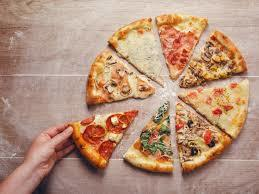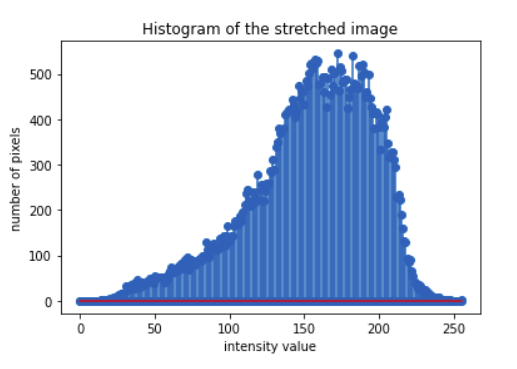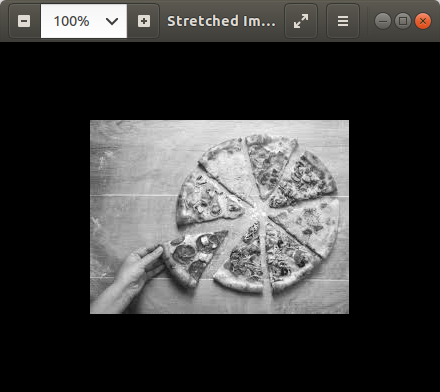# Histogram Plotting and stretching in Python (without using inbuilt function)

Histogram of a digital image with intensity levels in the range of 0 to L-1 is a discrete function –

```h(rk) = nk
where rk = kth intensity value
and no = number of pixels in the image with rk intensity value.```

If the image has M rows and N columns, then the total number of pixels in the image is MN. The normalized histogram is obtained as follows:

`p(rk) = nk/MN`

Sometimes if the image is a dark, light, or low contrast, then the histogram of that image might not have pixels with minimum intensity (0) or maximum intensity(255) or both respectively. In such cases, the histogram of that image can be stretched by applying the following transformation.

```Let a= maximum intensity level in the image
Let b= minimum intensity level in the image
Let rk= pixel value in the original image
Let sk= pixel value in the stretched image
constant= (255-0)/(a-b)

Then
sk= constant*rk```

Algorithm for Histogram plotting

• Obtain the size of the image. Let m=rows and n=columns
• For all the intensity levels rk= 0 to 255
• Traverse all the rows ‘m’ and columns ‘n’
• Count the number of pixels for each intensity level
• Plot the graph of intensity values versus number of pixels in each intensity value

Algorithm to stretch the Histogram

• Find the minimum intensity value in the image say ‘a’ and maximum intensity value say ‘b’
• obtain a constant c= (255-0)/(a-b)
• Multiply this constant with each pixel in the image to obtain histogram stretching.

Observations
It is observed that the dark input image improves after contrast stretching.

Below is the implementation.

Input Image:`# import cv2, numpy, matplotlib ` `import` `cv2 ` `import` `numpy as np ` `import` `matplotlib.pyplot as plt ` `  `  `     `  `# function to obtain histogram of an image ` `def` `hist_plot(img): ` `     `  `    ``# empty list to store the count  ` `    ``# of each intensity value ` `    ``count ``=``[] ` `     `  `    ``# empty list to store intensity  ` `    ``# value ` `    ``r ``=` `[] ` `     `  `    ``# loop to traverse each intensity  ` `    ``# value ` `    ``for` `k ``in` `range``(``0``, ``256``): ` `        ``r.append(k) ` `        ``count1 ``=` `0` `         `  `        ``# loops to traverse each pixel in  ` `        ``# the image  ` `        ``for` `i ``in` `range``(m): ` `            ``for` `j ``in` `range``(n): ` `                ``if` `img[i, j]``=``=` `k: ` `                    ``count1``+``=` `1` `        ``count.append(count1) ` `         `  `    ``return` `(r, count) ` ` `  ` `  `img ``=` `cv2.imread(``'food.jpeg'``, ``0``) ` ` `  `# To ascertain total numbers of rows and  ` `# columns of the image, size of the image ` `m, n ``=` `img.shape ` `r1, count1 ``=` `hist_plot(img) ` ` `  `# plotting the histogram ` `plt.stem(r1, count1) ` `plt.xlabel(``'intensity value'``) ` `plt.ylabel(``'number of pixels'``) ` `plt.title(``'Histogram of the original image'``) ` `  `  `# Transformation to obtain stretching ` `constant ``=` `(``255``-``0``)``/``(img.``max``()``-``img.``min``()) ` `img_stretch ``=` `img ``*` `constant ` `r, count ``=` `hist_plot(img_stretch) ` ` `  `# plotting the histogram ` `plt.stem(r, count) ` `plt.xlabel(``'intensity value'``) ` `plt.ylabel(``'number of pixels'``) ` `plt.title(``'Histogram of the stretched image'``) ` `  `  `# Storing stretched Image ` `cv2.imwrite(``'Stretched Image 4.png'``, img_stretch) `

Output:My Personal Notes arrow_drop_upCheck out this Author's contributed articles.

If you like GeeksforGeeks and would like to contribute, you can also write an article using contribute.geeksforgeeks.org or mail your article to contribute@geeksforgeeks.org. See your article appearing on the GeeksforGeeks main page and help other Geeks.

Please Improve this article if you find anything incorrect by clicking on the "Improve Article" button below.

Article Tags :

Be the First to upvote.

Please write to us at contribute@geeksforgeeks.org to report any issue with the above content.